• 机器学习深度学习高阶内容系列-Python实现凸优化求解器 CVXOPT是一个基于Python编程语言的凸优化的免费软件包。它可以与交互式Python解释器一起使用，通过执行Python脚本在命令行上使用，或者通过Python扩展模块集成...

机器学习深度学习高阶内容系列-Python实现凸优化求解器

CVXOPT是一个基于Python编程语言的凸优化的免费软件包。它可以与交互式Python解释器一起使用，通过执行Python脚本在命令行上使用，或者通过Python扩展模块集成到其他软件中。它的主要目的是通过构建Python广泛的标准库和Python作为高级编程语言的优势，使凸优化应用程序的软件开发变得简单明了。

CVXOPT用两个矩阵对象扩展了内置Python对象：一个用于密集矩阵的矩阵对象和一个用于稀疏矩阵的spmatrix对象。密集矩阵和稀疏矩阵一章介绍了这两种矩阵类型，以及为它们定义的算术运算和函数。 BLAS接口和稀疏线性方程 描述了几个用于密集和稀疏矩阵计算的库的接口。CVXOPT优化例程在Cone编程和建模章节中进行了描述。这些工具包括用Python编写的凸优化求解器、与其他一些优化库的接口，以及用于分段线性凸优化问题的建模工具。

CVXOPT 安装部署

展开全文• 对于一些非线性,多模型,多目标的函数优化问题,用其它优化方法较难求解,而用遗传算法则可以方便地得到较好的结果.用Matlab实现了函数优化的遗传算法源程序. 遗传算法的流程如图 1 所示。其主要组成部分包括：　 ​...

1 简介

函数优化是遗传算法的经典应用领域,也是遗传算法进行性能评价的常用算例.对于一些非线性,多模型,多目标的函数优化问题,用其它优化方法较难求解,而用遗传算法则可以方便地得到较好的结果.用Matlab实现了函数优化的遗传算法源程序.

几个与浮点数编码和二进制编码个体匹配的交叉运算：单点交叉，均匀交叉，算术交叉，两点交叉和多点交叉。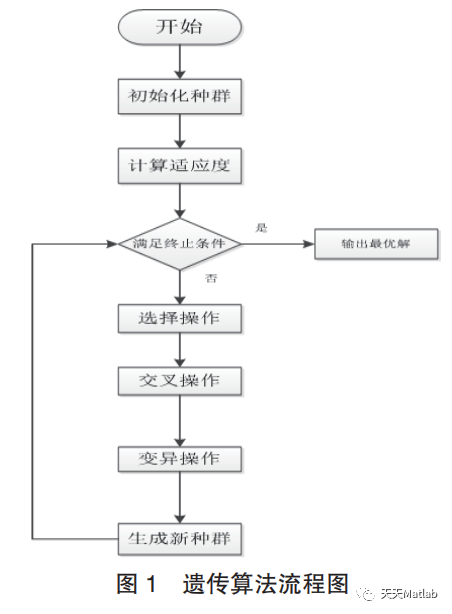2 部分代码

function varargout = yichuan(varargin)
% YICHUAN MATLAB code for yichuan.fig
%     YICHUAN, by itself, creates a new YICHUAN or raises the existing
%     singleton*.
%
%     H = YICHUAN returns the handle to a new YICHUAN or the handle to
%     the existing singleton*.
%
%     YICHUAN('CALLBACK',hObject,eventData,handles,...) calls the local
%     function named CALLBACK in YICHUAN.M with the given input arguments.
%
%     YICHUAN('Property','Value',...) creates a new YICHUAN or raises the
%     existing singleton*. Starting from the left, property value pairs are
%     applied to the GUI before yichuan_OpeningFcn gets called. An
%     unrecognized property name or invalid value makes property application
%     stop. All inputs are passed to yichuan_OpeningFcn via varargin.
%
%     *See GUI Options on GUIDE's Tools menu. Choose "GUI allows only one
%     instance to run (singleton)".
%

% Edit the above text to modify the response to help yichuan

% Begin initialization code - DO NOT EDIT
gui_Singleton = 1;
gui_State = struct('gui_Name',       mfilename, ...
'gui_Singleton',  gui_Singleton, ...
'gui_OpeningFcn', @yichuan_OpeningFcn, ...
'gui_OutputFcn',  @yichuan_OutputFcn, ...
'gui_LayoutFcn', [] , ...
'gui_Callback',   []);
if nargin && ischar(varargin{1})
gui_State.gui_Callback = str2func(varargin{1});
end

if nargout
[varargout{1:nargout}] = gui_mainfcn(gui_State, varargin{:});
else
gui_mainfcn(gui_State, varargin{:});
end
% End initialization code - DO NOT EDIT

% --- Executes just before yichuan is made visible.
function yichuan_OpeningFcn(hObject, eventdata, handles, varargin)
% This function has no output args, see OutputFcn.
% hObject   handle to figure
% eventdata reserved - to be defined in a future version of MATLAB
% handles   structure with handles and user data (see GUIDATA)
% varargin   command line arguments to yichuan (see VARARGIN)

% Choose default command line output for yichuan
handles.output = hObject;

% Update handles structure
guidata(hObject, handles);

% UIWAIT makes yichuan wait for user response (see UIRESUME)
% uiwait(handles.figure1);

% --- Outputs from this function are returned to the command line.
function varargout = yichuan_OutputFcn(hObject, eventdata, handles)
% varargout cell array for returning output args (see VARARGOUT);
% hObject   handle to figure
% eventdata reserved - to be defined in a future version of MATLAB
% handles   structure with handles and user data (see GUIDATA)

% Get default command line output from handles structure
varargout{1} = handles.output;

% --- Executes on button press in pushbutton1.
function pushbutton1_Callback(hObject, eventdata, handles)
% hObject   handle to pushbutton1 (see GCBO)
% eventdata reserved - to be defined in a future version of MATLAB
% handles   structure with handles and user data (see GUIDATA)
global x
%%%%%%%%%%%%%%%%%%%%%%%%%%%%%%%%%%%%%%%%%%%%%%%
%%%%%绘制题中函数图形%%%%%%%%%%%%%%%%%%%%%%%%%%%
x=linspace(-1,2);
y=10+x.*cos(5*pi*x);

axes(handles.axes1)
plot(x,y,'r')
title('函数曲线图')
xlabel('x')
ylabel('y')

% --- Executes on button press in pushbutton2.
function pushbutton2_Callback(hObject, eventdata, handles)
% hObject   handle to pushbutton2 (see GCBO)
% eventdata reserved - to be defined in a future version of MATLAB
% handles   structure with handles and user data (see GUIDATA)
global BitLength         %全局变量，计算如果满足求解精度至少需要编码的长度
global boundsbegin       %全局变量，自变量的起始点
global boundsend         %全局变量，自变量的终止点
=
end
Generation=Generation-1;%Generation加1、减1的操作是为了能记录各代中的最佳函数值xmax(Generation)
targetfunvalue=targetfun(xmax)
[Besttargetfunvalue,nmax]=max(targetfunvalue)
Bestpopulation=xmax(nmax)
%绘制经过遗传运算后的适应度曲线
axes(handles.axes2)
hand1=plot(1:Generation,ymax);
set(hand1,'linestyle','-','linewidth',1,'marker','*','markersize',8)
hold on;

hand2=plot(1:Generation,ymean);
set(hand2,'color','k','linestyle','-','linewidth',1, 'marker','h','markersize',8)
xlabel('进化代数');
ylabel('最大和平均适应度');
xlim([1 Generationnmax]);
legend('最大适应度','平均适应度');
box off;
hold off;

% --- Executes on button press in pushbutton3.
function pushbutton3_Callback(hObject, eventdata, handles)
% hObject   handle to pushbutton3 (see GCBO)
% eventdata reserved - to be defined in a future version of MATLAB
% handles   structure with handles and user data (see GUIDATA)
clearall;
close all;

3 仿真结果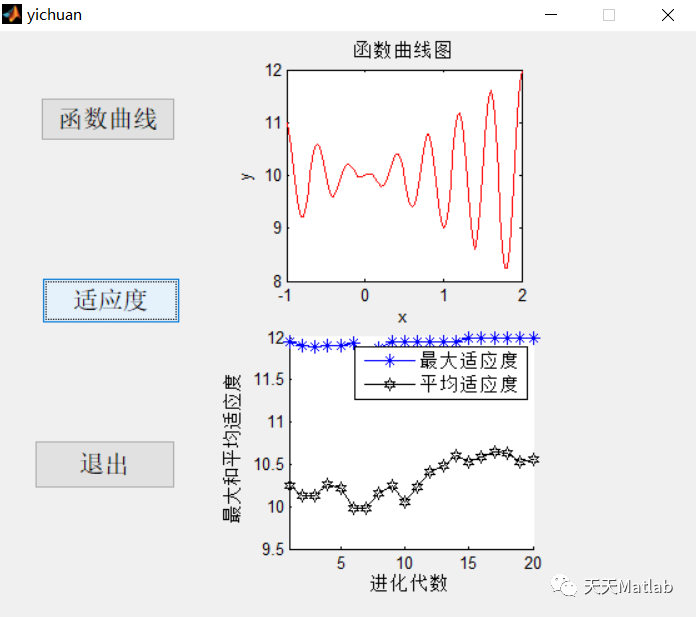4 参考文献

宋建萍. "函数优化的遗传算法代码实现." 软件导刊 12.002(2013):40-42.

部分理论引用网络文献，若有侵权联系博主删除。

5 MATLAB代码与数据下载地址

见博客主页

展开全文matlab 算法 开发语言
• 优化求解】基于粒子群算法求解多目标优化问题matlab源码 1 算法介绍 1.1 关于速度和位置 粒子群算法通过设计一种无质量的粒子来模拟鸟群中的鸟，粒子仅具有两个属性：速度和位置，速度代表移动的快慢，位置代表...

1 算法介绍

1.1 关于速度和位置

粒子群算法通过设计一种无质量的粒子来模拟鸟群中的鸟，粒子仅具有两个属性：速度和位置，速度代表移动的快慢，位置代表移动的方向。

鸟被抽象为没有质量和体积的微粒(点)，并延伸到N维空间，粒子i在N维空间的位置表示为矢量Xi＝(x1，x2，…，xN)，飞行速度表示为矢量Vi＝(v1，v2，…，vN)。每个粒子都有一个由目标函数决定的适应值(fitness value)，并且知道自己到目前为止发现的最好位置(pbest)和现在的位置Xi。这个可以看作是粒子自己的飞行经验。除此之外，每个粒子还知道到目前为止整个群体中所有粒子发现的最好位置(gbest)(gbest是pbest中的最好值)，这个可以看作是粒子同伴的经验。粒子就是通过自己的经验和同伴中最好的经验来决定下一步的运动。

2.2 速度和位置的更新

PSO初始化为一群随机粒子(随机解)。然后通过迭代找到最优解。在每一次的迭代中，粒子通过跟踪两个“极值”(pbest，gbest)来更新自己。在找到这两个最优值后，粒子通过下面的公式来更新自己的速度和位置。对于公式(1)：

公式(1)的第①部分称为【记忆项】，表示上次速度大小和方向的影响；

公式(1)的第②部分称为【自身认知项】，是从当前点指向粒子自身最好点的一个矢量，表示粒子的动作来源于自己经验的部分；

公式(1)的第③部分称为【群体认知项】，是一个从当前点指向种群最好点的矢量，反映了粒子间的协同合作和知识共享。粒子就是通过自己的经验和同伴中最好的经验来决定下一步的运动。

以上面两个公式为基础，再来看一个公式：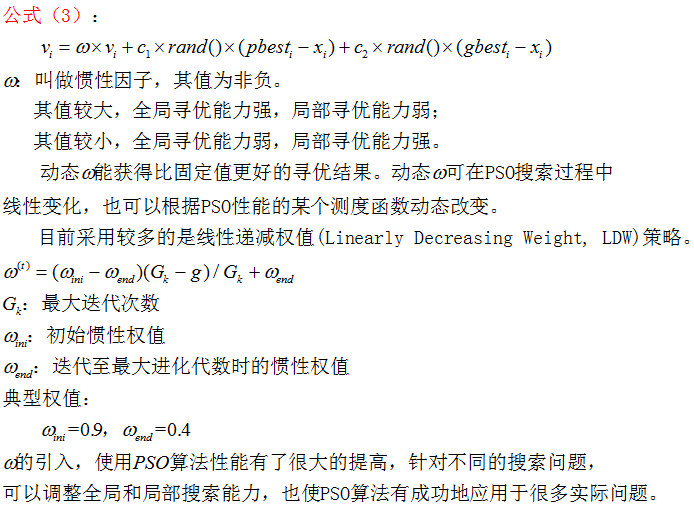公式(2)和 公式(3)被视为标准PSO算法。

1.3 标准PSO算法的流程

1）初始化一群微粒(群体规模为N)，包括随机位置和速度；

2）评价每个微粒的适应度；

3）对每个微粒，将其适应值与其经过的最好位置pbest作比较，如果较好，则将其作为当前的最好位置pbest；

4）对每个微粒，将其适应值与其经过的最好位置gbest作比较，如果较好，则将其作为当前的最好位置gbest；

5）根据公式(2)、(3)调整微粒速度和位置；

6）未达到结束条件则转第2）步。

迭代终止条件根据具体问题一般选为最大迭代次数Gk或(和)微粒群迄今为止搜索到的最优位置满足预定最小适应阈值。

## 4.2 PSO流程图解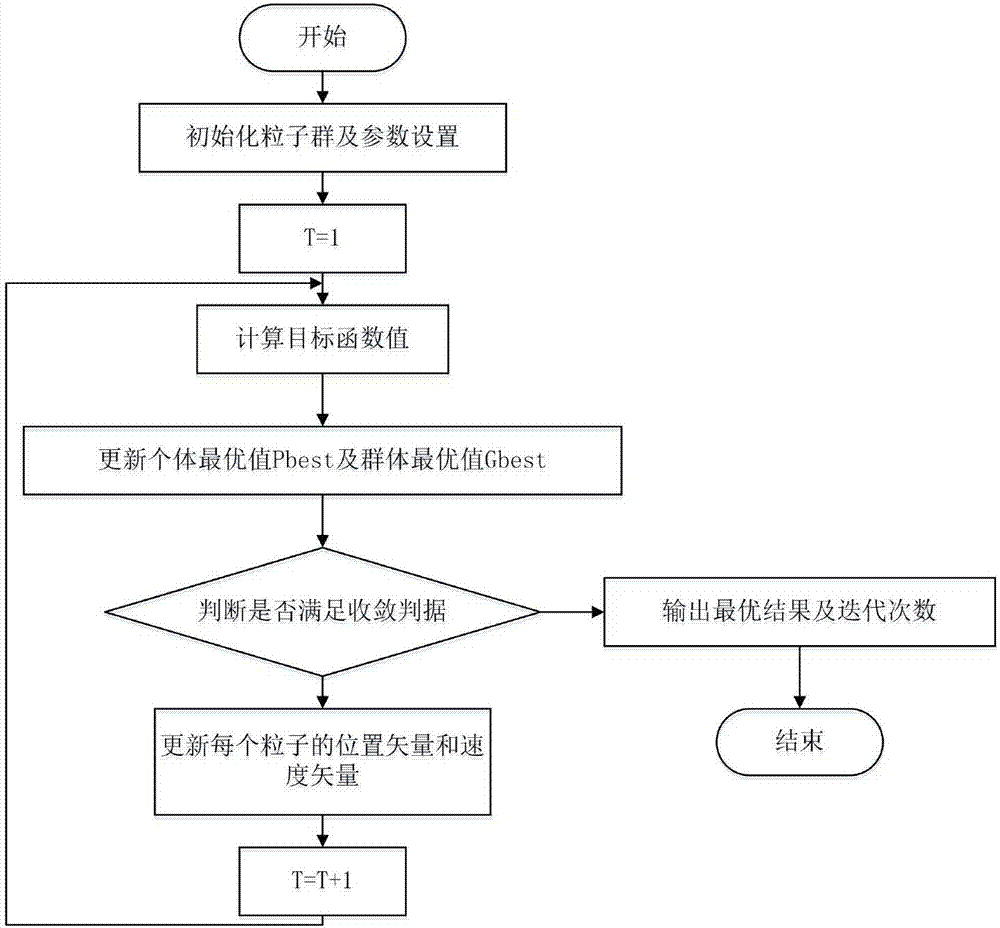2 部分代码

clc;
clear;
close all;
​
%% 问题定义，这里可以删除
​
TestProblem=1;   % Set to 1, 2, or 3
​
switch TestProblem
case 1
CostFunction=@(x) MyCost1(x);
nVar=50;
VarMin=0;
VarMax=1;

case 2
CostFunction=@(x) MyCost2(x);
nVar=3;
VarMin=-5;
VarMax=5;

case 3
CostFunction=@(x) MyCost3(x);
nVar=2;
VarMin=0;
VarMax=1;
end
​
VarSize=[1 nVar];
​
VelMax=(VarMax-VarMin)/10;
​
%% MOPSO 设置
nPop=500;   % Population Size
​
nRep=100;   % Repository Size
​
MaxIt=500;  % Maximum Number of Iterations
​
phi1=2.05;%学习因子
phi2=2.05;
phi=phi1+phi2;
chi=2/(phi-2+sqrt(phi^2-4*phi));
​
w=chi;              % Inertia Weight
wdamp=1;            % Inertia Weight Damping Ratio
c1=chi*phi1;        % Personal Learning Coefficient
c2=chi*phi2;        % Global Learning Coefficient
​
alpha=0.1;  % Grid Inflation Parameter
​
nGrid=10;   % Number of Grids per each Dimension
​
beta=4;     % Leader Selection Pressure Parameter
​
gamma=2;    % Extra (to be deleted) Repository Member Selection Pressure
​
%% 初始化
​
particle=CreateEmptyParticle(nPop);
​
for i=1:nPop
particle(i).Velocity=0;
particle(i).Position=unifrnd(VarMin,VarMax,VarSize); %unifrnd在[VarMin,VarMax]区间模拟VarSize的数组
% 初始化了自变量X的位置
particle(i).Cost=CostFunction(particle(i).Position); % 求解目标函数
particle(i).Best.Position=particle(i).Position;
particle(i).Best.Cost=particle(i).Cost;
end
​
particle=DetermineDomination(particle);
​
rep=GetNonDominatedParticles(particle);
​
rep_costs=GetCosts(rep);
G=CreateHypercubes(rep_costs,nGrid,alpha);
​
for i=1:numel(rep)
[rep(i).GridIndex rep(i).GridSubIndex]=GetGridIndex(rep(i),G);
end

%% MOPSO 主循环
​
for it=1:MaxIt
for i=1:nPop
​
particle(i).Velocity=w*particle(i).Velocity ...
+c1*rand*(particle(i).Best.Position - particle(i).Position) ...
+c2*rand*(rep_h.Position -  particle(i).Position);
​
particle(i).Velocity=min(max(particle(i).Velocity,-VelMax),+VelMax);
​
particle(i).Position=particle(i).Position + particle(i).Velocity;

flag=(particle(i).Position<VarMin | particle(i).Position>VarMax);    %非劣检查:flag
particle(i).Velocity(flag)=-particle(i).Velocity(flag);

particle(i).Position=min(max(particle(i).Position,VarMin),VarMax);
​
particle(i).Cost=CostFunction(particle(i).Position);
​
if Dominates(particle(i),particle(i).Best)
particle(i).Best.Position=particle(i).Position;
particle(i).Best.Cost=particle(i).Cost;

elseif ~Dominates(particle(i).Best,particle(i))
if rand<0.5
particle(i).Best.Position=particle(i).Position;
particle(i).Best.Cost=particle(i).Cost;
end
end
​
end

particle=DetermineDomination(particle);
nd_particle=GetNonDominatedParticles(particle);

rep=[rep
nd_particle];

rep=DetermineDomination(rep);
rep=GetNonDominatedParticles(rep);

for i=1:numel(rep)
[rep(i).GridIndex rep(i).GridSubIndex]=GetGridIndex(rep(i),G);
end

if numel(rep)>nRep
EXTRA=numel(rep)-nRep;
rep=DeleteFromRep(rep,EXTRA,gamma);

rep_costs=GetCosts(rep);
G=CreateHypercubes(rep_costs,nGrid,alpha);

end

%     disp(['Iteration ' num2str(it) ': Number of Repository Particles = ' num2str(numel(rep))]);

w=w*wdamp;
end
​
%% 结果
​
costs=GetCosts(particle);
rep_costs=GetCosts(rep);
​
figure;
​
plot(costs(1,:),costs(2,:),'b.');
hold on;
plot(rep_costs(1,:),rep_costs(2,:),'rx');
legend('Main Population','Repository');
​

3 仿真结果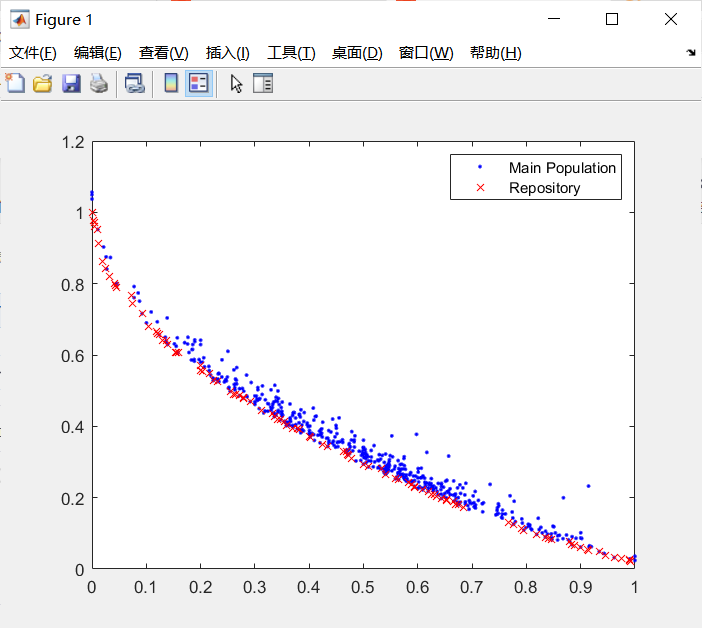4 参考文献

王越, 吕光宏. 改进的粒子群求解多目标优化算法[J]. 计算机技术与发展, 2014, 000(002):42-45.

5 代码下载展开全文matlab 算法 线性代数
• 标题优化工具包—无约束非线性优化求解器（fminunc） fminunc函数：求无约束多变量函数的最小值 一、fminunc总体介绍 语法： x = fminunc(fun,x0) x = fminunc(fun,x0,options) x = fminunc(problem) [x,fval] = ...

优化工具包—无约束非线性优化求解器（fminunc）

原创不易，路过的各位大佬请点个赞

fminunc函数：求无约束多变量函数的最小值一、fminunc总体介绍

语法：

x = fminunc(fun,x0)
x = fminunc(fun,x0,options)
x = fminunc(problem)
[x,fval] = fminunc(___)
[x,fval,exitflag,output] = fminunc(___)

求解如下目标函数的最小值
min ⁡ x f ( x ) \min_xf(x)
其中 f ( x ) f(x) 目标损失函数，即优化函数。 x x 为变量，fminunc求解器面向多变量函数，因此 x x 可以是向量和矩阵。与fminbnd求解器不同，它的变量必须是单变量。此外，fminunc 适用于无约束非线性问题。如果需要优化的问题有约束，通常使用 fmincon。

x = fminunc(fun,x0) 在点 x0 处开始并尝试求 fun 中描述的函数的局部最小值 x。点 x0 可以是标量、向量或矩阵。

二、fminunc求解器的具体用法

下面具体介绍fminunc求解器的用法

1）x = fminunc(fun,x0,options) 使用 options 中指定的优化选项最小化 fun。使用 optimoptions 可设置这些选项（后面介绍options）;

2）x = fminunc(problem) 求 problem 的最小值，它是 problem 中所述的一个结构体，描述一个优化问题；

3）[x,fval] = fminunc(___) 对上述任何语法，返回目标函数 fun 在解 x 处的值；

4）[x,fval,exitflag,output] = fminunc(___) 还返回描述 fminunc 的退出条件的值 exitflag，以及提供优化过程信息的结构体 output。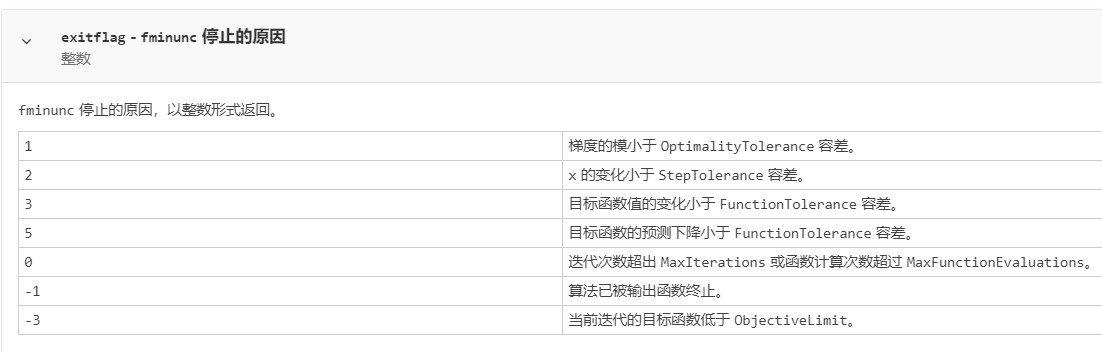~grad - fun 在解 x 处的梯度。
~hessian - fun 在解 x 处的 Hessian 矩阵。请参阅 fminunc Hessian 矩阵。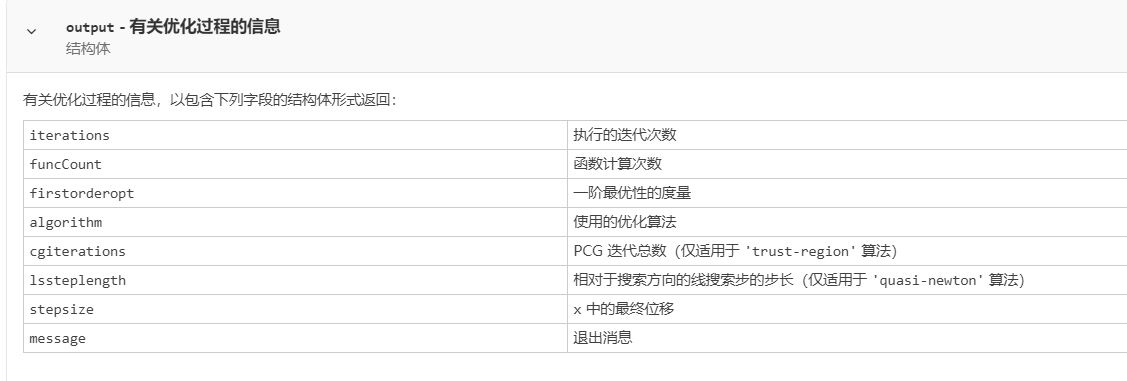三、举例：最小化多项式

目标函数：
f ( x ) = 3 x 1 2 + 2 x 2 x 2 + x 2 2 − 4 x 1 + 5 x 2 f(x)=3x_1^2+2x_2x_2+x_2^2-4x_1+5x_2
其中 x = [ x 1 , x 2 ] x=[x_1,x_2] 为一个二维向量。
代码：
给定初始值x0=[1,1];

fun = @(x)3*x(1)^2 + 2*x(1)*x(2) + x(2)^2 - 4*x(1) + 5*x(2);
x0 = [1,1];
[x,fval] = fminunc(fun,x0)

求解结果：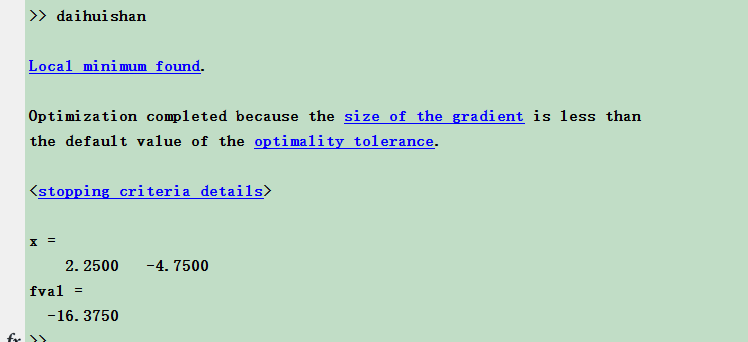目标函数的三维网格图：

fun = @(x) 3*x(1)^2 + 2*x(1)*x(2) + x(2)^2 - 4*x(1) + 5*x(2);
x0 = [1,1];
[x,fval] = fminunc(fun,x0)
xmin=x(1);ymin=x(2);zmin=fval;

syms x y
z=3*x^2 + 2*x*y + y^2 - 4*x + 5*y;
ezsurf(z,[-20,20],30);hold on;
plot3(xmin,ymin,zmin,'rp','markersize',30,'MarkerFaceColor','r')   %标记一个黑色的圆点
text(xmin,ymin,zmin,[' xmax=',num2str(xmin),char(10),'  y=',num2str(ymin),char(10),'  z=',num2str(zmin)]);   %标出坐标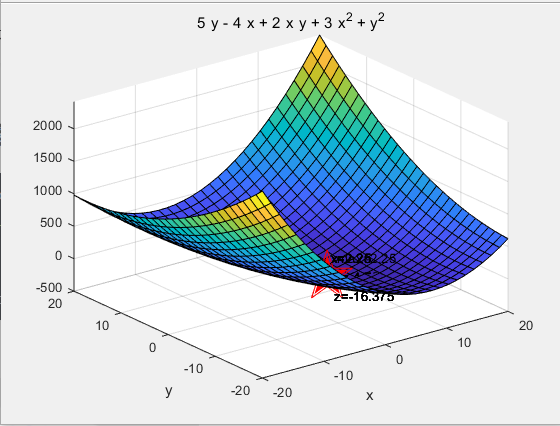当然也可以输出x处的梯度信息:

fun = @(x) 3*x(1)^2 + 2*x(1)*x(2) + x(2)^2 - 4*x(1) + 5*x(2);
x0 = [1,1];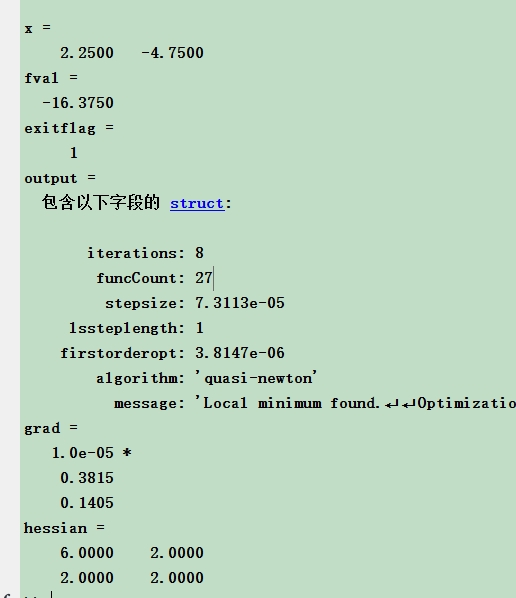四、举例—获取最佳目标函数值

求非线性函数最小值的位置和该最小值处的函数值。目标函数是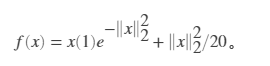fun = @(x)x(1)*exp(-(x(1)^2 + x(2)^2)) + (x(1)^2 + x(2)^2)/20;
x0 = [1,2];

结果如下

x = -0.6691 0.0000
fval = -0.4052
exitflag =
1 output = 包含以下字段的 struct:
iterations: 9
funcCount: 42
stepsize: 2.9345e-04
lssteplength: 1
firstorderopt: 8.0839e-07
algorithm: ‘quasi-newton’
message: ‘Local minimum found.↵↵Optimization completed because the size of the gradient is less than↵the default value of the
optimality tolerance.↵↵Stopping criteria details:↵↵Optimization
completed: The first-order optimality measure, 6.891345e-07, is less
↵than options.OptimalityTolerance = 1.000000e-06.↵↵Optimization Metric
OptimalityTolerance = 1e-06 (default)’
1.0e-06 *
0.8084
0.6817
hessian =
1.8998 -0.0000 -0.0000 0.9552
三维网格图如下：

close all;
fun = @(x)x(1)*exp(-(x(1)^2 + x(2)^2)) + (x(1)^2 + x(2)^2)/20;
x0 = [1,2];

figure
xmin=x(1);ymin=x(2);zmin=fval;
syms x y
z=x*exp(-(x^2 + y^2)) + (x^2 + y^2)/20;
ezsurf(z,[-20,20],30);hold on;
plot3(xmin,ymin,zmin,'rp','markersize',30,'MarkerFaceColor','r')   %标记一个黑色的圆点
text(xmin,ymin,zmin,[' xmax=',num2str(xmin),char(10),'  y=',num2str(ymin),char(10),'  z=',num2str(zmin)]);   %标出坐标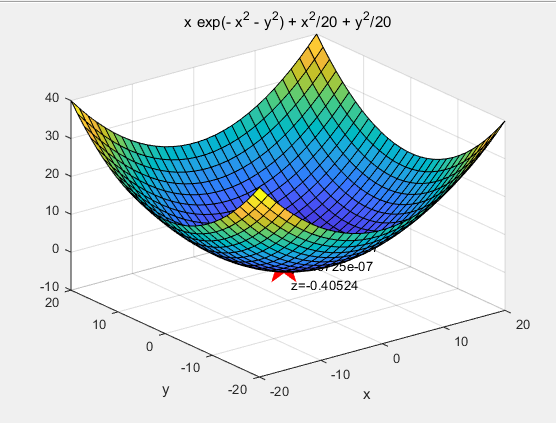画出等高线

close all
figure
X=-20:0.1:20;
[x,y]=meshgrid(X);
z=x.*exp(-(x.^2 + y.^2)) + (x.^2 + y.^2)./20;
meshc(x,y,z)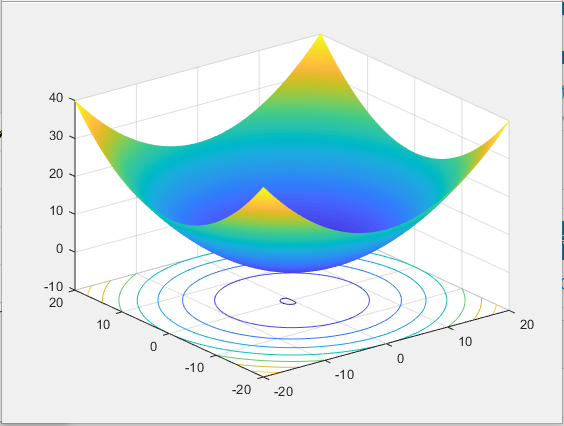五、检查求解过程（options 设置）

优化选项，指定为 optimoptions 的输出或 optimset 等返回的结构体。

语法：

options = optimoptions(@fminunc,'Algorithm','quasi-newton');

其中’Algorithm’设置优化工具包用什么优化算法求解问题，其中fminunc函数可以用拟牛顿法和信赖域算法。
设置fminunc采用拟牛顿法求解：其中该函数’Algorithm’的默认值为’quasi-newton’

options = optimoptions(@fminunc,'Algorithm','quasi-newton');

quasi-newton 算法使用具有三次线搜索过程的 BFGS 拟牛顿法。这种拟牛顿法使用 BFGS公式来更新 Hessian 矩阵的逼近。您可以通过将 HessUpdate 选项设置为 ‘dfp’（并将 Algorithm 选项设置为 ‘quasi-newton’）来选择逼近逆 Hessian 矩阵的 DFP公式。您可以通过将 HessUpdate 设置为 ‘steepdesc’（并将 Algorithm 设置为 ‘quasi-newton’）来选择最陡下降法，尽管此设置通常效率不高。

设置fminunc采用信赖域算法求解：必须自己提供目标函数的梯度，不建议使用。

options = optimoptions(@fminunc,'Algorithm','trust-region');

trust-region 算法要求您在 fun 中提供梯度，并使用 optimoptions 将 SpecifyObjectiveGradient 设置为 true。此算法是一种子空间信赖域方法，基于  和  中所述的内部反射牛顿法。每次迭代都涉及使用预条件共轭梯度法 (PCG) 来近似求解大型线性方程组。

用法：

fun = @(x)x(1)*exp(-(x(1)^2 + x(2)^2)) + (x(1)^2 + x(2)^2)/20;
x0 = [1,2];

其它options的参数表如下：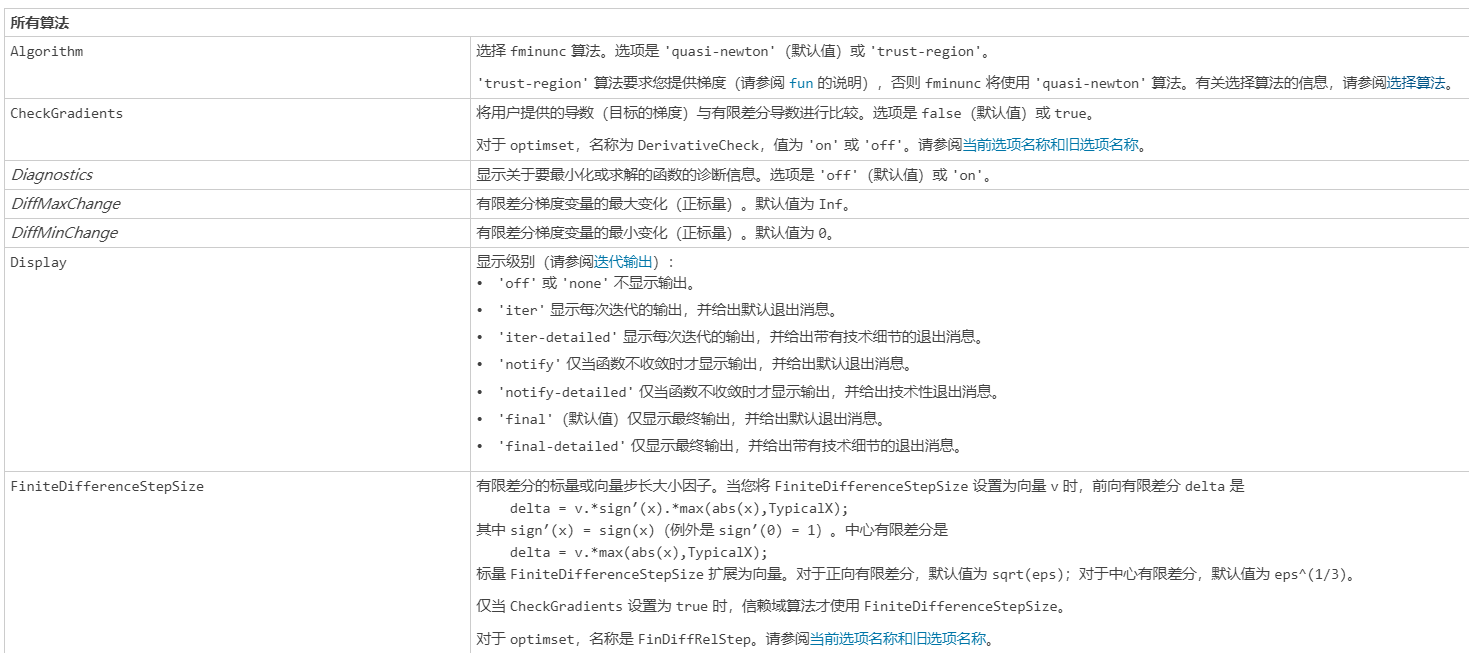六、信赖域法实例（fminunc求解器trust-region设置）

fminunc 有两种算法：
‘quasi-newton’（默认值）
‘trust-region’
在命令行中使用 optimoptions 设置 Algorithm 选项。

建议
如果您的目标函数包括梯度，请使用 ‘Algorithm’ = ‘trust-region’，并将 SpecifyObjectiveGradient 选项设置为 true。
否则，请使用 ‘Algorithm’ = ‘quasi-newton’。

6.1 信赖域实例1

考虑以下优化问题：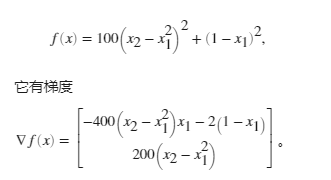% Calculate objective f
f = 100*(x(2) - x(1)^2)^2 + (1-x(1))^2;

if nargout > 1 % gradient required
g = [-400*(x(2)-x(1)^2)*x(1) - 2*(1-x(1));
200*(x(2)-x(1)^2)];
end
end

创建使用目标函数的梯度的选项。此外，将算法设置为 ‘trust-region’。

将初始点设置为 [-1,2]。然后调用 fminunc。

x0 = [-1,2];
x = fminunc(fun,x0,options)

结果

Local minimum found.

Optimization completed because the size of the gradient is less than
the value of the optimality tolerance.
x = 1×2

1.0000    1.0000

6.2 信赖域实例2：使用问题结构体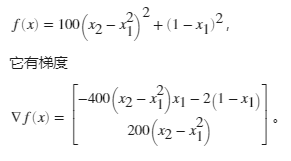% Calculate objective f
f = 100*(x(2) - x(1)^2)^2 + (1-x(1))^2;

if nargout > 1 % gradient required
g = [-400*(x(2)-x(1)^2)*x(1)-2*(1-x(1));
200*(x(2)-x(1)^2)];
end
end

将算法设置为 ‘trust-region’。

创建包括初始点 x0 = [-1,2] 的问题结构体。有关此结构体中的必填字段，

problem.options = options;
problem.x0 = [-1,2];
problem.solver = 'fminunc';

求解

x = fminunc(problem)

结果

Local minimum found.

Optimization completed because the size of the gradient is less than
the value of the optimality tolerance.
x = 1×2

1.0000    1.0000

原创不易，路过的各位大佬请点个赞

展开全文matlab
• 从https://solver.damo.alibaba.com求解器SDK下载和安装 (aliyun.com)https://solver.damo.alibaba.com中下载 Windows 64-bit/x86MindOpt发行版（win64-x86-0.14.0），双击安装文件，根据指引安装即可。 二、配...python 阿里云
• 我需要帮助将我的问题表述为Python中的约束优化问题。在假设我有一个PandasDataFrame的视频，这些视频包含以下列id, views, scoreid是每个视频的唯一标识views是视频的观看次数score是计算视频质量分数的函数f的输出...
• 考虑到模型的简便性以及求解的简便性，所以本设计不考虑公交公司车辆的的固定费用。 1.2乘客出行成本分析 本设计中乘客出行成本主要考虑乘客的候车时间最短。当一天内乘客的平均候车时间最短即认为乘客的出行本...人工智能 python 机器学习 算法 java
• 一、获取代码方式 获取代码方式1： 完整代码已上传我的资源：【多目标优化求解】基于matlab金鹰算法求解多目标优化问题【含Matlab源码 188期】 获取代码方式2： 通过订阅紫极神光博客付费专栏，凭支付凭证，私信博主...matlab 算法 神经网络
• MATLAB多目标约束优化求解该极值问题可以考虑fmincon(解，也可以用ga()求解(遗传算法)于给出的目数 F 缺具y1(x1,x2,x3)、y2(x1,x2,x3)、y3(x1,x2,x3)的表达式。所以无法帮助你。matlab中优化问题，关于不等式约束...
• 1 简介 平衡 优 化 器 ( equilibrium optimizer，EO) 是 Afshin ... "一种基于改进平衡优化器算法的光伏电池参数识别方法.". 部分理论引用网络文献，若有侵权联系博主删除。 5 MATLAB代码与数据下载地址 见博客主页matlab 算法 开发语言
• 模拟退火算法(Simulated Annealing，SA)的思想最早由Metropolis等人于1953年提出：Kirkpatrick于1983年第一次使用模拟退火算法求解组合最优化问题 。模拟退火算法是一种基于MonteCarlo迭代求解策略的随机寻优算法...matlab 模拟退火算法 算法
• 本文将介绍R中可用于投资组合优化的不同求解器。 通用求解器 通用求解器可以处理任意的非线性优化问题，但代价可能是收敛速度慢。 默认包 包stats（默认安装的基本R包）提供了几个通用的优化程序。 optimize()...R语言 优化求解器 优化 非线性规划
• 粒子群优化(PSO)是一种基于群体智能的数值优化算法，由社会心理学家James Kennedy和电气工程师Russell Eberhart于1995年提出。自PSO诞生以来，它在许多方面都得到了改进，这一部分将介绍基本的粒子群优化算法原理和...matlab 算法 机器学习
• 算法具有调节参数较少、易于实现等优点，成为近几年进化计算领域的重要算法和研究热点之一，已被成功应用于杠杆结构优化、电力系统的无功优化调度和无人机航线规划等许多问题的优化求解当中． 2 部分代码 ...算法 matlab 开发语言
• 在电脑上安装VS . 2,在电脑安装scip 3.直接pip install pyscipopt 时会报错 4.找到安装后的scip，把scip、src都拷贝进去 5.重新安装、 6.案例优化器 机器学习
• 其中， 模拟蚁群觅食过程的蚁群优化算法(Ant Colony Optimization， A CO) 和模拟鸟群运动方式的粒子群算法(ParticleS warm Optimization，PSO) 是两种最主要的群智能算法。 蚁群算法是一种源于大自然生物世界的新的...matlab 算法
• 狮群优化算法（Loin Swarm Optimization, LSO），是于2018年提出的一种新型智能优化算法。基于狮群中狮王、母狮及幼狮的自然分工,模拟狮王守护、母狮捕猎、幼狮跟随3种群智能行为,提出群体智能算法——狮群算法.算法...算法 matlab 开发语言
• LocalSolver创新性地结合了精确算法和启发式算法，在基准测试中多次击败了Gurobi或IBM ILOG Cplex等传统求解器。除了提供快速和可扩展的全局优化解决方案，我们的团队为您提供专门的和响应性的支持，使用LocalSolver...人工智能 机器学习
• 阿基米德优化算法：一种解决优化问题的新元启发式算法 现实世界中的数值优化问题的难度和复杂性日益增加，这需要有效的优化方法。迄今为止，已经引入了各种元启发式方法，但只有少数在研究界得到认可。本文提出了一...matlab 算法 线性代数
• Q: Gurobi优化求解变量时能设置各优化变量的猜想最优初始值吗？ 可以通过 Var.Start 属性设置初始解，而且可以同时设置多组初始解，初始解可以不完整，可以不可行，gurobi 会根据初始解信息，自动凑齐全部变量，...
• 一、算法原理之前我们了解过的算法大部分都是无约束优化问题，其算法有：黄金分割法，牛顿法，拟牛顿法，共轭梯度法，单纯性法等。但在实际工程问题中，大多数优化问题都属于有约束优化问题。惩罚函数法就可以将约束...
• 使用yalmip求解鲁棒优化前言鲁棒优化简介yalmip实操求解优化问题示例代码结果总结前言记录一下早期夭折的研究想法，想使用鲁棒优化(robust optimization，RO)解决整数变量的资源分配问题。目标函数和约束条件都很...
• 鸟群算法( bird swarm alogrithm，BSA) 是由 Meng 等人于 2015 年提出的一种基于鸟群行为的群智能优化算法，其思想源于鸟群的飞行、觅食和警戒三个主要群体行为，BSA算法依赖飞行行为跳出局部最优进行全局搜索，通过...算法 matlab 开发语言
• ChOA通过模拟攻击黑猩猩、驱赶黑猩猩、拦截黑猩猩和追逐黑猩猩4类黑猩猩协同狩猎行为来达到求解问题的目的。与其他算法相比， ChOA具有收敛速度快、寻优精度高等特点。 (1)驱赶和追逐猎物。 在黑猩猩狩猎过程中，...matlab 算法 线性代数
• 粒子群优化(PSO)是一种基于群体智能的数值优化算法，由社会心理学家James Kennedy和电气工程师Russell Eberhart于1995年提出。自PSO诞生以来，它在许多方面都得到了改进，这一部分将介绍基本的粒子群优化算法原理和...matlab 算法 机器学习
• 1 秃鹰搜索优化算法 秃鹰遍布于北美洲地区, 飞行中视力敏锐, 观察能力优秀. 以捕食鲑鱼为例, 秃鹰首先会基于个体和种群到鲑鱼的浓度来选择搜索空间, 朝一个特定区域飞行; 其次在选定搜索空间内搜索水面, 直到发现...matlab 图像处理 开发语言
• 一、获取代码方式 获取代码方式1： 完整代码已上传我的资源：【多目标优化求解】基于matlab灰狼优化算法求解多目标优化问题 【含Matlab源码 007期】 获取代码方式2： 通过订阅紫极神光博客付费专栏，凭支付凭证，私...matlab 算法 机器学习
• 完整代码已上传我的资源：【多目标优化求解】基于matlab遗传优化萤火虫算法求解多目标优化问题【含Matlab源码 1484期】 备注：开通CSDN会员，仅只能免费获得1份代码（有效期为开通日起，三天内有效）； 订阅紫极神光...matlab 算法 开发语言
• 该算法是一种基于迭代的优化技术,初始化为一组随机解,然后 通过迭代搜寻最优解,且在最优解周围通过随机飞行产生局部新解,加强了局部搜索。与其他算法相比,BA 在准确性和有效性方面远优于其他算法,且没有许多参数要...matlab 算法 机器学习...